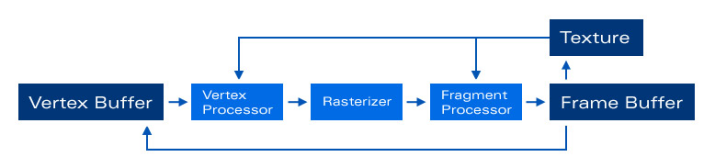# instantreality 1.0

Keywords:
tutorial, X3D, world, rendering
Author(s): Yvonne Jung
Date: 2007-02-05

Summary: This tutorial shows how to write a simple shader in X3D.

Uniform parameters can be set via the dynamic field mechanism: For each uniform variable needed, a field or an exposedField with the same name and data type should be defined. This has to be done in the ComposedShader node. In order to define textures (uniform variables of any sampler type), an exposedField of type SFInt32 referring to the appropriate texture unit has to be defined (in case of the following example this is the first one, i.e. unit 0, because no MultiTexture is used).

When writing a geometry shader, there are three other fields for defining input and output primitive types as well as the maximum number of vertices to be generated in the shader: geometryInputType, geometryOutputType and geometryVerticesOut.

Note: Generic vertex attribute parameters are currently not supported. If they are needed (e.g.tangents), they can be encoded in the texCoord or color field of the geometry node.

### Code: A basic shader appearance

```	Shape {
appearance Appearance {
texture ImageTexture {
url "testImage.jpg"
}
exposedField SFInt32 tex0    0
exposedField SFVec3f diffuse .7 .7 .7

parts [
type "vertex"
}
type "fragment"
}
]
}
}
geometry Sphere	{}
}

```

If you want to use CG for your shader programs, you have to replace the ComposedShader node by a ProgramShader node with its language field set to "cg". Additionally the parts field has to be replaced by the programs field and the ShaderPart nodes by ShaderProgram nodes.Image: Program Stages for Shader Model 3.0 graphics hardware.

This example shows, how a simple glsl shader implementation is done within X3D. For additionally demonstrating how to access a texture, the diffuse color is taken from an earth texture. In order to ensure the correct mapping between the X3D field defining the texture unit and the sampler uniform definition, both variables must have the same name, which - in case of our example - is earthTex. By the way, the same goes for all other uniform variables likewise.

### Code: Uniform parameters

```	Appearance {
texture ImageTexture {
url "earth.jpg"
}
exposedField SFInt32 earthTex 0
exposedField SFFloat ambient  .2
exposedField SFVec3f specular .7 .7 .7

parts [
# ...
]
}
}

```In the vertex shader the incoming vertex position is transformed with the current modelview-projection matrix with the help of the ftransform function. After that all varying parameters, which are required by the subsequent fragment shader and interpolated across the primitive during rasterization (like gl_TexCoord and the user defined ones for normal, eye vector and light vector) are calculated.

### Code:

```	DEF vs ShaderPart {
type "vertex"
url "
varying vec3 lightVec;
varying vec3 eyeVec;
varying vec3 normalVec;

void main(void)
{
gl_Position = ftransform();

gl_TexCoord = gl_MultiTexCoord0;
normalVec = gl_Normal;

vec4 eyePos = gl_ModelViewMatrixInverse * vec4(0., 0., 0., 1.);
eyeVec = eyePos.xyz - gl_Vertex.xyz;

vec4 lightPos = gl_ModelViewMatrixInverse * vec4(gl_LightSource.position.xyz, 1.0);
lightVec = lightPos.xyz - gl_Vertex.xyz;
}
"
}

```

The fragment shader realizes the Blinn-Phong lighting model, without attenuation factor (the complete formula is depicted in the following image). After texture access all vectors are normalized and the final fragment color is calculated. A good introductory text to glsl shader programming is the so-called Orange Book. Another application domain for shader programming is imaging; in the files section you will find examples for both.Image: Blinn-Phong lighting formula without attenuation, the operator symbol defines the component-wise product.

### Code:

```	DEF fs ShaderPart {
type "fragment"
url "
uniform sampler2D earthTex;

uniform vec3 specular;
uniform float ambient;

varying vec3 lightVec;
varying vec3 eyeVec;
varying vec3 normalVec;

void main(void)
{
vec3 texCol = texture2D(earthTex, gl_TexCoord.st).rgb;

lightVec = normalize(lightVec);
eyeVec = normalize(eyeVec);
normalVec = normalize(normalVec);
vec3 halfVec = normalize( eyeVec + lightVec );

float ndotl = max( dot( lightVec, normalVec ), 0.0 );
float ndoth = (ndotl > 0.0) ? pow(max( dot( halfVec, normalVec ), 0.0 ), 128.) : 0.0;

vec3 color = 0.2*ambient + ndotl*texCol + ndoth*specular;

gl_FragColor = vec4(color, 1.0);
}
"
}

```

## Using HDR Images

### Code: Using an hdr image of type OpenEXR

```	ImageCubeMapTexture {
repeatS FALSE
repeatT FALSE
repeatR FALSE
internalFormat "rgba16f"
url "StageEnvCube.exr"
}

```

Geometry shaders (an example is shown below, additionally you find a simple test in the files section) require to set some additional fields:

• geometryOutputType defines the output primitive type for the geometry shader, possible values are "auto", "points", "lines", "line_strip", "triangles", "triangle_strip";
• geometryVerticesOut sets the maximum number of vertices to be generated by the geometry shader, which is important for performance.

### Code:

```	ComposedShader {
geometryInputType  "points"
geometryOutputType "triangle_strip"
geometryVerticesOut 16

exposedField SFInt32 dataFieldTex 0
exposedField SFInt32 edgeTableTex 1
exposedField SFInt32 triTableTex 2
exposedField SFVec3f dataStep 0.02 0.02 0.04
exposedField SFFloat isolevel 0.2
#[...]

parts [
type "VERTEX"
url "TestG80_VS.glsl"
}
type "GEOMETRY"
url "TestG80_GS.glsl"
}
type "FRAGMENT"
url "TestG80_FS.glsl"
}
]
}

```
Files: